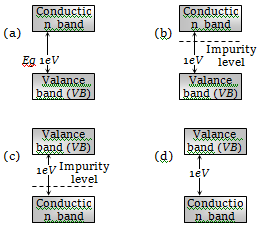Which of the following has negative temperature coefficient of resistance
(a) Copper               (b) Aluminium
(c) Iron                   (d) Germanium

Concept Questions :-

Types of semiconductors
High Yielding Test Series + Question Bank - NEET 2020

Difficulty Level:

In semiconductors at a room temperature

(a) The valence band is partially empty and the conduction band is partially filled

(b) The valence band is completely filled and the conduction band is partially filled

(c) The valence band is completely filled

(d) The conduction band is completely empty

Concept Questions :-

Energy band theory
High Yielding Test Series + Question Bank - NEET 2020

Difficulty Level:

Regarding a semiconductor which one of the following is wrong

(a) There are no free electrons at room temperature

(b) There are no free electrons at 0 K

(c) The number of free electrons increases with rise of temperature

(d) The charge carriers are electrons and holes

Concept Questions :-

Types of semiconductors
High Yielding Test Series + Question Bank - NEET 2020

Difficulty Level:

Which of the following statements is true for an N-type semi-conductor

(a) The donor level lies closely below the bottom of the conduction band

(b) The donor level lies closely above the top of the valence band

(c) The donor level lies at the halfway mark of the forbidden energy gap

(d) None of above

Concept Questions :-

Types of semiconductors
High Yielding Test Series + Question Bank - NEET 2020

Difficulty Level:

Choose the correct statement

(a) When we heat a semiconductor its resistance increases
(b) When we heat a semiconductor its resistance decreases
(c) When we cool a semiconductor to 0 K then it becomes super conductor
(d) Resistance of a semiconductor is independent of temperature

Concept Questions :-

Types of semiconductors
High Yielding Test Series + Question Bank - NEET 2020

Difficulty Level:

Intrinsic semiconductor is electrically neutral. Extrinsic semiconductor having large number of current carriers would be

(a) Positively charged

(b) Negatively charged

(c) Positively charged or negatively charged depending upon the type of impurity that has been added

(d) Electrically neutral

Concept Questions :-

Types of semiconductors
High Yielding Test Series + Question Bank - NEET 2020

Difficulty Level:

If ${\mathrm{n}}_{\mathrm{e}}$ and ${\mathrm{v}}_{\mathrm{d}}$ be the number of electrons and drift velocity in a semiconductor. When the temperature is increased

(a) ${\mathrm{n}}_{\mathrm{e}}$ increases and ${\mathrm{v}}_{\mathrm{d}}$ decreases
(b) ${\mathrm{n}}_{\mathrm{e}}$ decreases and ${\mathrm{v}}_{\mathrm{d}}$ increases
(c) Both ${\mathrm{n}}_{\mathrm{e}}$ and ${\mathrm{v}}_{\mathrm{d}}$ increases
(d) Both ${\mathrm{n}}_{\mathrm{e}}$ and ${\mathrm{v}}_{\mathrm{d}}$ decreases

Concept Questions :-

Types of semiconductors
High Yielding Test Series + Question Bank - NEET 2020

Difficulty Level:

The energy gap of silicon is 1.14 eV. The maximum wavelength at which silicon will begin absorbing energy is

(a) 10888 Å                    (b) 1088.8 Å
(c) 108.88 Å                   (d) 10.888 Å

Concept Questions :-

Energy band theory
High Yielding Test Series + Question Bank - NEET 2020

Difficulty Level:

Which of the following energy band diagram shows the N-type semiconductorConcept Questions :-

Energy band theory
High Yielding Test Series + Question Bank - NEET 2020

Difficulty Level:

The mobility of free electrons is greater than that of free holes because

1. They carry negative charge

2. They are light

3. They mutually collide less

4. They require low energy to continue their motion

Concept Questions :-

Types of semiconductors